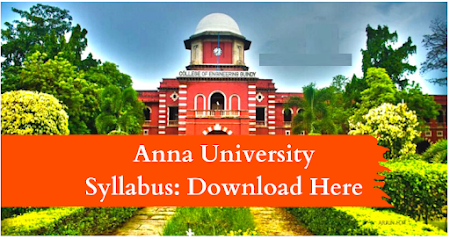### MA8551       ALGEBRA AND NUMBER THEORY   L    T    P    C  4    0    0    4

OBJECTIVES:  To introduce the basic notions of groups, rings, fields which will then be used to solve related problems.   To introduce and apply the concepts of rings, finite fields and polynomials.    To understand the basic concepts in number theory  To examine the key questions in the Theory of Numbers.  To give an integrated approach to number theory and abstract algebra, and provide a firm basis for further reading and study in the subject.

UNIT I          GROUPS AND RINGS                                                                                               12 Groups : Definition - Properties - Homomorphism - Isomorphism - Cyclic groups - Cosets - Lagrange's theorem. Rings: Definition - Sub rings - Integral domain - Field - Integer modulo n - Ring homomorphism.

UNIT II     FINITE FIELDS AND POLYNOMIALS                                                                     12 Rings - Polynomial rings - Irreducible polynomials over finite fields - Factorization of polynomials over finite fields.

UNIT III     DIVISIBILITY THEORY AND CANONICAL DECOMPOSITIONS                   12 Division algorithm – Base - b representations – Number patterns – Prime and composite numbers – GCD – Euclidean algorithm – Fundamental theorem of arithmetic – LCM.

UNIT IV     DIOPHANTINE EQUATIONS AND CONGRUENCES                                          12  Linear Diophantine equations – Congruence‘s – Linear Congruence‘s - Applications: Divisibility tests - Modular exponentiation-Chinese remainder theorem – 2 x 2 linear systems.

UNIT V       CLASSICAL THEOREMS AND MULTIPLICATIVE FUNCTIONS                    12 Wilson‘s theorem – Fermat‘s little theorem – Euler‘s theorem – Euler‘s  Phi  functions – Tau and Sigma functions.

TOTAL: 60 PERIODS
OUTCOMES: Upon successful completion of the course, students should be able to:   Apply the basic notions of groups, rings, fields which will then be used to solve related problems.   Explain the fundamental concepts of advanced algebra and their role in modern             mathematics and applied contexts.   Demonstrate accurate and efficient use of advanced algebraic techniques.          Demonstrate their mastery by solving non - trivial problems related to the concepts, and by proving simple theorems about the, statements proven by the text.  Apply  integrated  approach to number theory and abstract algebra, and provide a firm basis for further reading and study in the subject.

TEXTBOOKS:
1. Grimaldi, R.P and Ramana, B.V., "Discrete and Combinatorial Mathematics", Pearson           Education, 5th Edition, New Delhi, 2007.  2. Koshy, T., ―Elementary Number Theory with Applications‖, Elsevier Publications,  New Delhi, 2002.

REFERENCES:
1. Lidl, R. and Pitz, G, "Applied Abstract Algebra", Springer Verlag, New Delhi, 2nd Edition, 2006. 2. Niven, I., Zuckerman.H.S., and Montgomery, H.L., ―An Introduction to Theory of Numbers‖, John Wiley and Sons , Singapore, 2004.
3. San Ling and Chaoping Xing, ―Coding Theory – A first Course‖, Cambridge Publications, Cambridge, 2004.